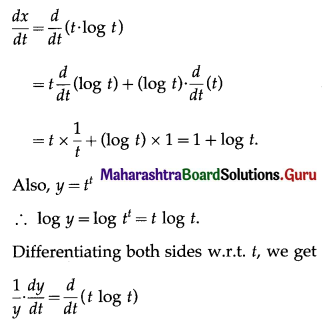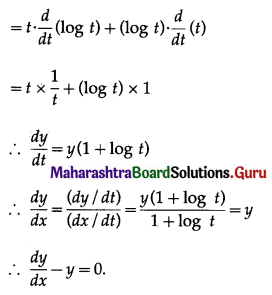# Maharashtra Board 12th Commerce Maths Solutions Chapter 3 Differentiation Ex 3.5

Balbharati Maharashtra State Board 12th Commerce Maths Solution Book Pdf Chapter 3 Differentiation Ex 3.5 Questions and Answers.

## Maharashtra State Board 12th Commerce Maths Solutions Chapter 3 Differentiation Ex 3.5

1. Find $$\frac{d y}{d x}$$ if:

Question 1.
x = at2, y = 2at
Solution:
x = at2, y = 2at
Differentiating x and y w.r.t. t, we get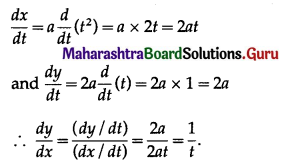Question 2.
x = 2at2, y = at4
Solution: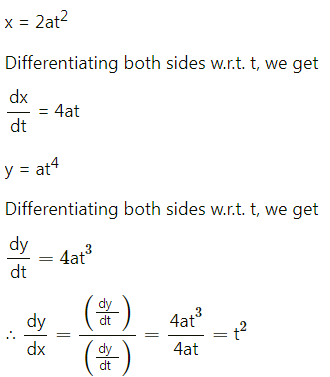Question 3.
x = e3t, y = e(4t+5)
Solution:
x = e3t, y = e(4t+5)
Differentiating x and y w.r.t. t, we get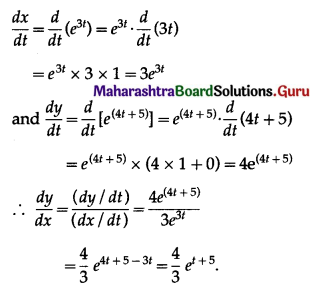2. Find $$\frac{d y}{d x}$$ if:

Question 1.
x = $$\left(u+\frac{1}{u}\right)^{2}$$, y = $$(2)^{\left(u+\frac{1}{u}\right)}$$
Solution:
x = $$\left(u+\frac{1}{u}\right)^{2}$$, y = $$(2)^{\left(u+\frac{1}{u}\right)}$$ ……(1)
Differentiating x and y w.r.t. u, we get,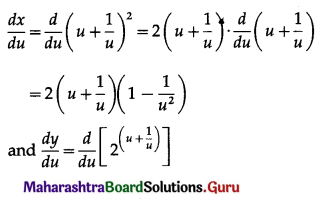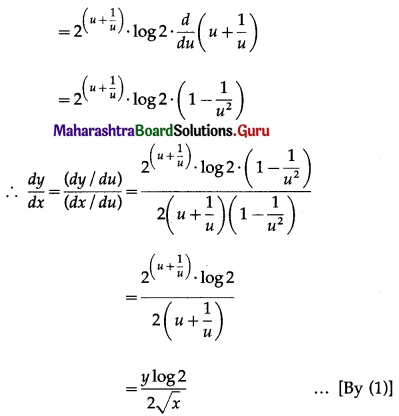Question 2.
x = $$\sqrt{1+u^{2}}$$, y = log(1 + u2)
Solution:
x = $$\sqrt{1+u^{2}}$$, y = log(1 + u2) ……(1)
Differentiating x and y w.r.t. u, we get,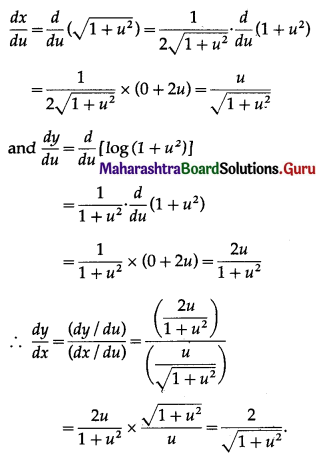Question 3.
Differentiate 5x with respect to log x.
Solution:
Let u = 5x and v = log x
Then we want to find $$\frac{d u}{d v}$$
Differentiating u and v w.r.t. x, we get
$$\frac{d u}{d x}=\frac{d}{d x}\left(5^{x}\right)=5^{x} \cdot \log 5$$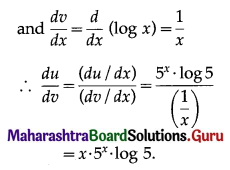3. Solve the following:

Question 1.
If x = $$a\left(1-\frac{1}{t}\right)$$, y = $$a\left(1+\frac{1}{t}\right)$$, then show that $$\frac{d y}{d x}$$ = -1
Solution: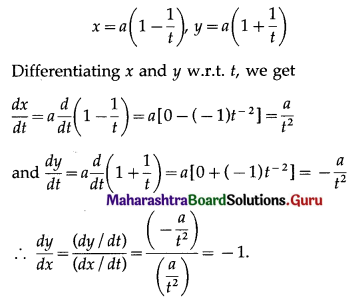Question 2.
If x = $$\frac{4 t}{1+t^{2}}$$, y = $$3\left(\frac{1-t^{2}}{1+t^{2}}\right)$$, then show that $$\frac{d y}{d x}=-\frac{9 x}{4 y}$$
Solution: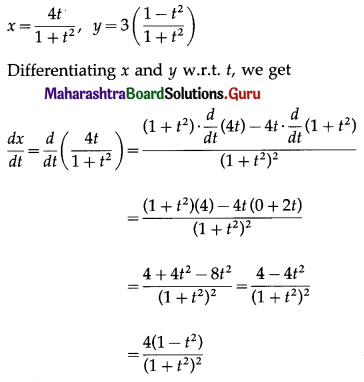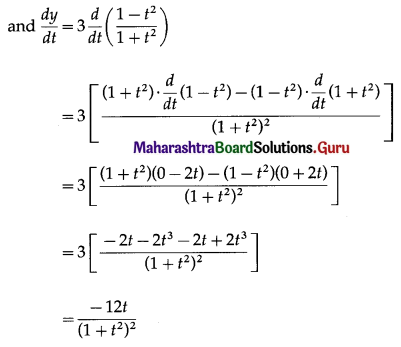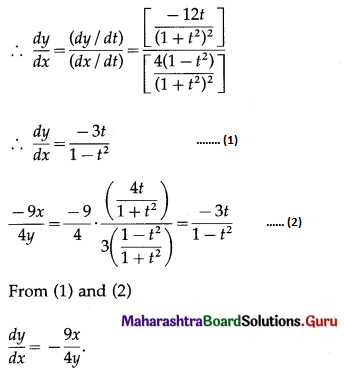Question 3.
If x = t . log t, y = tt, then show that $$\frac{d y}{d x}$$ – y = 0.
Solution:
x = t log t
Differentiating w.r.t. t, we get